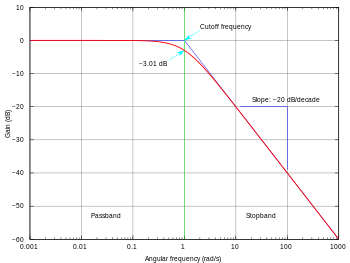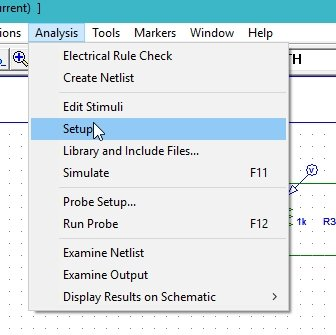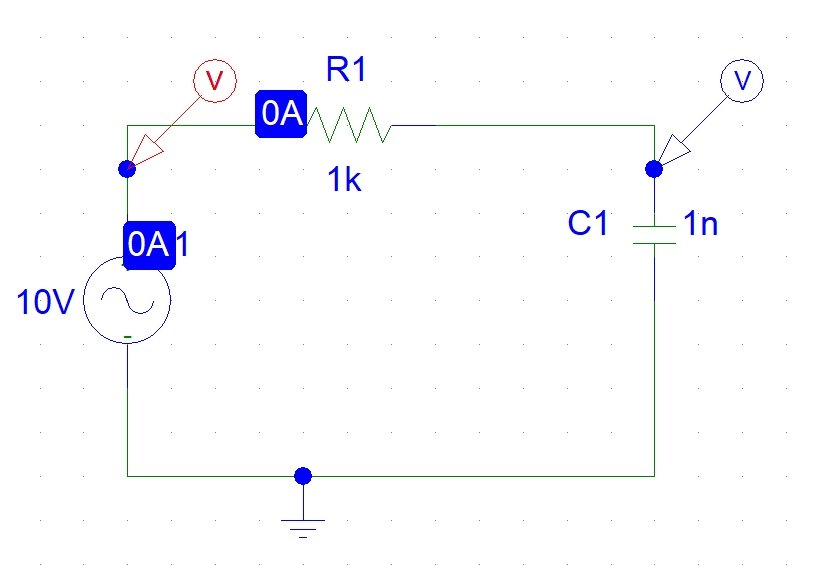# Low pass filter design and simulation using Pspice : tutorial 9

In this tutorial I will explain you the working of a low pass filter. The purpose of a low pass filter is to allow only the lower frequencies to pass through the circuit and block the higher frequencies. At the start a brief and concise introduction of filters specifically low pass filter along with them bode plots are provided with the explanation of the output they will show. After that the circuits is simulated using PSPICE and you are provided with the step by step approach to simulate a circuit and the result are compared with the theoretical discussion provided (which should be same). At the end of the tutorial you are provided with an exercise to do it by yourself, and in the next tutorials I will assume that you have done those exercises and I will not explain the concept regarding them

### Introduction to low pass filter

A low pass filter is such a filter which only allows frequencies with lower magnitude to pass through them and block the higher frequencies as the name suggests. The limit of the frequencies up to which the circuit allows is known as critical frequency and is given by the formula,

This frequency is present at -3db of the maximum magnitude or 0.707 or 70% of the maximum magnitude. The output plot in frequency domain or the bode plots of a simple low pass filter is given in the figure below,Figure 1: Low pass filter

### How to design and simulate low pass filter in PSpice

Figure 2: Opening schematic

Figure 3: Saving schematic

Figure 4: getting new part

Figure 5: Placing ac input

Figure 5: Placing capacitor

Figure 6: Placing resistor

Figure 7: Placing ground

Figure 8: Placed components

Figure 9: Draw wire

Figure 10: Complete circuit diagram

Figure 11: Voltage marker

Figure 12: Voltage marker placed

Figure 13: Input source attributes

• The adjustable attributes in the AC supply is only the magnitude of the AC voltage and all other attributes should be left as it is. The DC attribute is used to adjust the offset of the ac voltage which is not need in this experiment hence left as 0.
• Set the value of the capacitor according to the requirement of your circuit by double clicking on the component and changing the value to 3u and then clicking save attr but I am leaving the value in this experiment as it is.
• Next step is to adjust the properties of the simulations in order to produce the graph of the voltage at the marker. Click on analysis and then click on Setup as shown in the figure belowFigure 14: Simulation setup

Figure 15: AC sweep properties

Figure 17: Labeling the wire

Figure 18: Simulation

Figure 19: Output of simulation

• From the output it is obvious that the circuit simulated is a low pass filter. Above is shown the bode plot (frequency domain plot) of the circuit. At lower frequencies the circuit is allowing the voltage to pass through it and at higher frequencies it drops down to zero. Also the results are in accordance with the theoretical introduction.
• Connect another voltage marker at the input of the AC source to the see the AC sweep of the source along with that of the capacitor as shown in the figure below,Figure 20: Voltage maker at input

Figure 21: Output displayed with input

• The green line at the top represents the input AC sweep of the AC source connect. The straight line represents that the input source is constant (neither increasing nor decreasing with time) unlike that of the capacitor voltage. In the frequency domain we can say that the input source is allowing all the frequencies to pass hence straight.

Exercise:

• Try do the frequency domain analysis of any of the circuits we have done previously.

<< Previous tutorial                                                      Next tutorial>>